Test: Probability and Statistics - 4

# Test: Probability and Statistics - 4

Test Description

## 20 Questions MCQ Test Topic-wise Tests & Solved Examples for IIT JAM Mathematics | Test: Probability and Statistics - 4

Test: Probability and Statistics - 4 for Mathematics 2023 is part of Topic-wise Tests & Solved Examples for IIT JAM Mathematics preparation. The Test: Probability and Statistics - 4 questions and answers have been prepared according to the Mathematics exam syllabus.The Test: Probability and Statistics - 4 MCQs are made for Mathematics 2023 Exam. Find important definitions, questions, notes, meanings, examples, exercises, MCQs and online tests for Test: Probability and Statistics - 4 below.
Solutions of Test: Probability and Statistics - 4 questions in English are available as part of our Topic-wise Tests & Solved Examples for IIT JAM Mathematics for Mathematics & Test: Probability and Statistics - 4 solutions in Hindi for Topic-wise Tests & Solved Examples for IIT JAM Mathematics course. Download more important topics, notes, lectures and mock test series for Mathematics Exam by signing up for free. Attempt Test: Probability and Statistics - 4 | 20 questions in 60 minutes | Mock test for Mathematics preparation | Free important questions MCQ to study Topic-wise Tests & Solved Examples for IIT JAM Mathematics for Mathematics Exam | Download free PDF with solutions
 1 Crore+ students have signed up on EduRev. Have you?
Test: Probability and Statistics - 4 - Question 1

### The probability that a number in {1, 2,..., 1001} is divisible by 7 or 11 or both, is

Detailed Solution for Test: Probability and Statistics - 4 - Question 1

Number of integers divisible by 7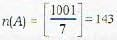Number of integers divisible by 11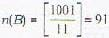Number of integers divisible by both 7 and 11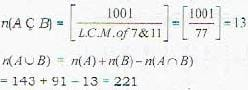Required probability of = 221/1001 Ans.

Test: Probability and Statistics - 4 - Question 2

### If A and B are two events, the probability that exactly one of them occurs is

Detailed Solution for Test: Probability and Statistics - 4 - Question 2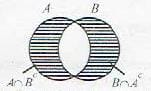Prob. that exactly one of them occurs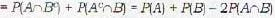Test: Probability and Statistics - 4 - Question 3

### A number is chosen from each of the two sets {1 , 2 , 3, 4, 5, 6 , 7. 8 , 9} and {1, 2 . 3, 4, 5, 6 , 7, 8 , 9}. If p1 denotes the probability that the sum of the two numbers be 10 and p2 the probability that their sum be 8, then (p1 + p2] is

Detailed Solution for Test: Probability and Statistics - 4 - Question 3

Sum is 10 if 1 + 9, 2 + 8,...9 + 1 is taken 9 cases.
sum is 8 if 1 + 7, 2 + 6,...7 + 1 is laken 7 cases.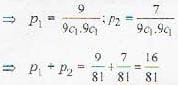Test: Probability and Statistics - 4 - Question 4

The probability that a non-leap year should have 53 Sunday is

Detailed Solution for Test: Probability and Statistics - 4 - Question 4

If year starts with Sunday it will end on Sunday, but year can start on any one of 7 days
Hence prob. = 1/7

Test: Probability and Statistics - 4 - Question 5

A coin is biased so that the probability of head = 1/4. The coin is tossed five times. The probability of obtaining two heads and three tails with heads occurring in succession is

Detailed Solution for Test: Probability and Statistics - 4 - Question 5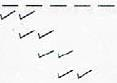Two consecutive head can appear in 4 ways remaining places goes to tail, so require probability is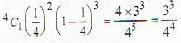Test: Probability and Statistics - 4 - Question 6

15 coupons are numbered 1,2 ..... 15. Seven coupons are selected at random, one at a time, with replacement. The probability that the largest number appearing on a selected coupon is 9, is

Detailed Solution for Test: Probability and Statistics - 4 - Question 6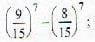; as numbers from 1 to 9 is selected 7 times and we subtract the case in which number 1 to 8 is selected all 7 times.

Test: Probability and Statistics - 4 - Question 7

Let A and B be any two arbitrary’ events, then, which of the following is true?

Detailed Solution for Test: Probability and Statistics - 4 - Question 7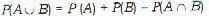⇒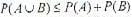Test: Probability and Statistics - 4 - Question 8

A die is loaded in such a way that each odd number is twice as likely to occur as each even number. If E is the event that a number greater than or equal to 4 occurs on a single toss of the die, then P (E) is

Detailed Solution for Test: Probability and Statistics - 4 - Question 8

Let probability of occurence of even number be k, then probability of occurence of odd number will be 2 k.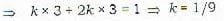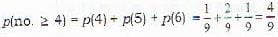Test: Probability and Statistics - 4 - Question 9

The probability that a person tossing three fair coins will get together all heads or all tails for the second time on the 5th toss is

Detailed Solution for Test: Probability and Statistics - 4 - Question 9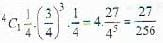Test: Probability and Statistics - 4 - Question 10

The probabilities that three men hit a target are 1/6, 1/4, 1/3 respectively. Each shoots once the target. What is the probability that exactly one of them hits the target?

Detailed Solution for Test: Probability and Statistics - 4 - Question 10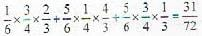Test: Probability and Statistics - 4 - Question 11

Let X, Y. Z be three independent normal variables N(0, 1). Then E(X - Y + Z)2] is

Detailed Solution for Test: Probability and Statistics - 4 - Question 11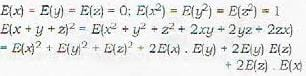As, x, y, z are independent.
= 1 + 1 + 1 + 0 + 0 + 0 = 3

Test: Probability and Statistics - 4 - Question 12

A probability distribution must possess

Detailed Solution for Test: Probability and Statistics - 4 - Question 12

Every probability distribution possess distribution function but mean, mode and moment generaLinig function may not exist.

Test: Probability and Statistics - 4 - Question 13

In a Poisson distribution

Detailed Solution for Test: Probability and Statistics - 4 - Question 13

R.V.X follows poisson distribution if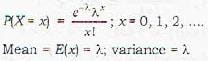Test: Probability and Statistics - 4 - Question 14

For a frequency distribution of marks in Mathematics for 100 students, the average was found to be 80. Later on it was discovered that. 4 8 was misread as 84. The correct mean is

Detailed Solution for Test: Probability and Statistics - 4 - Question 14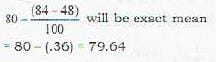Test: Probability and Statistics - 4 - Question 15

For a binomial distribution, the mean is (15/4) and the variance is (15/16). The value of p is

Detailed Solution for Test: Probability and Statistics - 4 - Question 15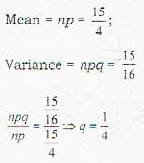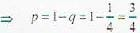Test: Probability and Statistics - 4 - Question 16

Following is the distribution of marks in Statistics obtained by 50 students :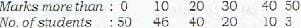If 60% of students passes the test, then what is the minimum marks obtained by a candidate who has passed?

Detailed Solution for Test: Probability and Statistics - 4 - Question 16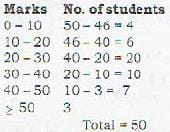60 % students have passed, so 50 x 60% = 30 students have passed.
Total 30 students fall in class 20 - 30 whose mid value is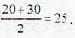Hence answer is 25.

Test: Probability and Statistics - 4 - Question 17

Telephone calls come into an exchange according to a Poisson process with 5 calls per minute on the average. The probability that no call will come in during the two minute period 10 A.M. to 10:02 A.M. on a particular day is

Detailed Solution for Test: Probability and Statistics - 4 - Question 17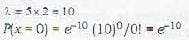Test: Probability and Statistics - 4 - Question 18

The coefficient of correlation between X and Y for the following bivariate distribution is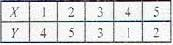Detailed Solution for Test: Probability and Statistics - 4 - Question 18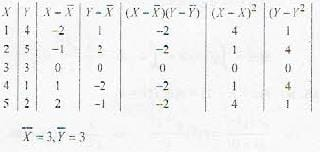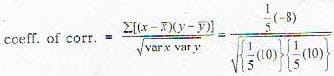= -4/5

Test: Probability and Statistics - 4 - Question 19

X1, X2, .... Xn are independent observations on the random variable X with distribution function F(x). Then the distribution function of Y = Max (X1, X2, ..., Xn) is

Detailed Solution for Test: Probability and Statistics - 4 - Question 19

[F(x)]n

Test: Probability and Statistics - 4 - Question 20

Telephone calls come into an exchange according to a Poisson process with 5 calls per minute on the average. The probability that no call will come in during the two-minute period 10 A.M. to 10:02 A.M. on a particular day is

Detailed Solution for Test: Probability and Statistics - 4 - Question 20

λ = 5 x 2 = 10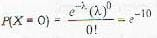## Topic-wise Tests & Solved Examples for IIT JAM Mathematics

27 docs|150 tests
 Use Code STAYHOME200 and get INR 200 additional OFF Use Coupon Code
Information about Test: Probability and Statistics - 4 Page
In this test you can find the Exam questions for Test: Probability and Statistics - 4 solved & explained in the simplest way possible. Besides giving Questions and answers for Test: Probability and Statistics - 4, EduRev gives you an ample number of Online tests for practice

## Topic-wise Tests & Solved Examples for IIT JAM Mathematics

27 docs|150 tests